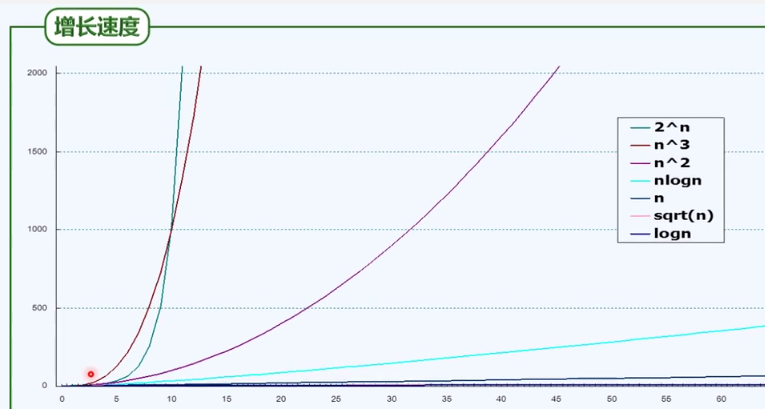# big-o

## 一、用途

度量算法的相对复杂度 ，也就是算法相对复杂度的一种表示方法。

## 二、原理

big-o主要从两个方面常数项可以忽略，低次项可以忽略入手。因为我们的目的是反映出算法的相对复杂度，对于一个计算公式来说，我们要简化对它的复杂度表示方法。比如：当n>>2时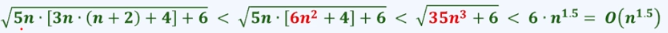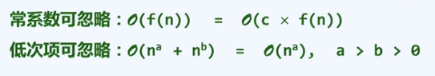a,b大于0

## 三、分类

太多的理论总是太空泛，所以例子会更加有助于我们理解知识点并且加深印象。总体来说，我们可以把big-o看做一把直尺，直尺上面有各种复杂度标度来帮助我们实现对算法复杂度的度量。

### 1、O(1)

最简单的复杂度记为：O(1),对应的就是常数，因为常数是最简单的，不用算直接就是结果。在实际算法中肯定是不可能的，我们把不含转向（循环，递归，调用）的顺序执行的算法复杂度称为O(1)；这个过程也要区分一些伪递归，伪循环等。

### 2、O(logan$log_an$)

对于对数多项式，常底数和常次数幂都是可以省略的。比如：常底数可以用换底公式，logan=logablogbn$log_an=log_ab*log_bn$而常数logab$log_ab$可以忽略掉，所以大O记号就是Ologbn$O（log_bn）$。同样道理，对于常次数幂，lnnc=clnn$lnn^c=c*lnn$其中c可以忽略所以大o记号就是O（lnn$lnn$）。明白这两点进而，ln321n+ln145n=Oln321n)$ln^{321}n+ln^{145}n=O（ln^{321}n)$对于对数多项式$\forall$c>0,logn=o(nc)$logn=o(n^c)$是无限接近于O(1)的。
因此对于以上两种类型O(1)和O(logan$log_an$)，复杂度都是比较小的，算法是比较有效的。

### 3、O(nc$n^c$)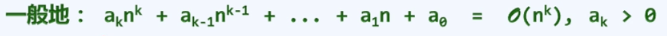我们之前也说过这个多项式处理方法，常数项忽略，低次幂项忽略。大部分编程题都是介于O(n)和O(n^2)的复杂度。这种算法的复杂度还是可以被我们所接受的。

### 4、O(2n$2^n$)

举个例子，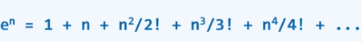可以看出这种指数函数可怕的计算量，对于这种情况我们是不能忍受的。一般从O(nc$n^c$)到O(2n$2^n$)是一个分水岭，前面的O(1)、O(logan$log_an$)和O(nc$n^c$)都可以接受。O(2n$2^n$)的复杂度就是不可接受的。

## 四、总结

下面以一张图彰显各种函数在大O记号下的增长速度，其中纵轴为复杂度，横轴为n。